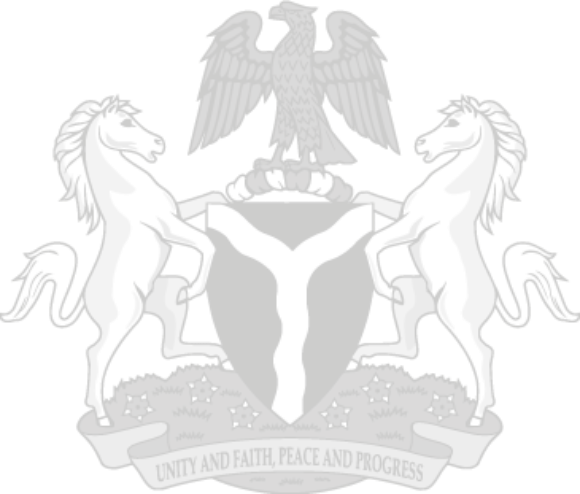## Summary

In the third quarter of 2022, Nigeria’s real GDP at basic prices grew by 2.25% on a year-on-year basis, showing a lower growth rate compared to the corresponding quarter of 2021. Growth of the Q4 of 2022 GDP remained positive at 3.52%, though lower compared to the Q4 of 2021 (3.98%). However, relative to Q3 and Q4 of 2021, the performances in 2022 indicate a decrease of 1.78% points in the Q3 of 2022 growth rate and a fall of 0.47% points in Q4 of 2022. Annual growth in 2022 was 3.10% compared to 3.40% recorded in 2021.
1. Household Consumption Expenditure, in Q3 and Q4 of 2022 grew by -5.83% and -12.47% in real terms, year-on-year. The growth rates in Q3 and Q4 of 2022 were lower than the rates recorded in Q3 and Q4 of 2021. The annual growth rate in 2022 stood at -4.07% compared to 25.65% in 2021. Government Consumption Expenditure recorded growth rates of -9.38% and -13.77% in Q3 and Q4 of 2022 respectively, on a year-on-year basis, higher, compared to -39.51% and -16.76% in Q3 & Q4 of 2021. Overall, government consumption expenditure grew by -9.91% in 2022.
2. Net Exports recorded a growth rate since Q2 of 2022, a departure from a negative growth rate in the first quarter of 2022. Net exports grew in real terms in Q3 and Q4 2022 by 74.11% and 161.66% respectively. These rates were higher than -38.27% and 1.35% recorded in Q3, and Q4 of 2021 respectively. On an annual basis, the growth rate of net exports in 2022 stood at 84.39% relative to -55.77% in 2021.
3. National Disposable Income grew by 1.08% and 4.98% in Q3 and Q4 of 2022 respectively, but recorded growth of -1.46% and 2.76% in Q3 and Q4 of 2021 respectively on a year-on-year basis in real terms. The growth of National Disposable Income became positive since Q4 of 2021, showing a better performance in Q3 & Q4 of 2022 relative to Q3 and Q4 of 2021. However, the annual growth rate in 2022 stood at 2.45% relative to -2.54% in 2021.
4. Compensation of Employees in the Q3 and Q4 of 2022 grew by 4.28%, and 3.28% respectively in real terms on a year-on-year basis. These growth rates were lower than the 14.54% and 11.79% recorded in Q3 and Q4 of 2021 respectively. The annual growth rate in 2022 stood at 4.41% relative to 13.68% in 2021.

Data source: National Bureau of Statistics

### INFOGRAPHICS### Portal & Apps